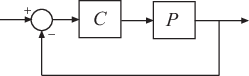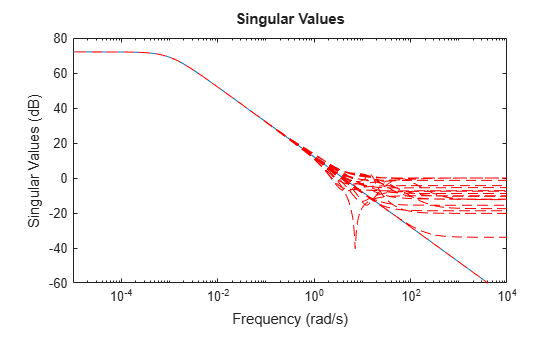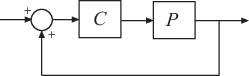# ncfmargin

Calculate normalized coprime stability margin of plant-controller feedback loop

## Syntax

``[marg,freq] = ncfmargin(P,C)``
``[marg,freq] = ncfmargin(P,C,sign)``
``[marg,freq] = ncfmargin(___,tol)``

## Description

example

````[marg,freq] = ncfmargin(P,C)` returns the normalized coprime stability margin of the multivariable feedback loop consisting of a controller `C` in negative feedback with a plant `P`:The normalized coprime robust stability margin (also called the gap metric stability margin) is an indication of robustness to unstructured perturbations. Values greater than 0.3 generally indicate good robustness margins.```
````[marg,freq] = ncfmargin(P,C,sign)` specifies the sign of the feedback connection assumed for the margin calculation. By default, `sign = -1`. Set `sign = +1` for positive-feedback interconnection.`[marg,freq] = ncfmargin(___,tol)` calculates the normalized coprime factor metric with the specified relative accuracy.```

## Examples

collapse all

Consider an unstable first-order plant, `p`, stabilized by high-gain and low-gain controllers, `cL` and `cH`.

```p = tf(4,[1 -0.001]); cL = 1; cH = 10;```

Compute the stability margin of the closed-loop system with the low-gain controller.

`[margL,~] = ncfmargin(p,cL)`
```margL = 0.7069 ```

Similarly, compute the stability margin of the closed-loop system with the high-gain controller.

`[margH,~] = ncfmargin(p,cH)`
```margH = 0.0995 ```

The closed-loop systems with low-gain and high-gain controllers have normalized coprime stability margins of about 0.71 and 0.1, respectively. This result indicates that the closed-loop system with low-gain controller is more robust to unstructured perturbations than the system with the high-gain controller.

To observe this difference in robustness, construct an uncertain plant, `punc`, that has additional unmodeled dynamics at high frequency compared to the nominal plant.

```punc = p + ultidyn('uncstruc',[1 1],'Bound',1); sigma(p,punc,'r--')```Calculate the robust stability of the closed-loop systems formed by the uncertain plant and each controller.

`[stabmargL,~] = robstab(feedback(punc,cL))`
```stabmargL = struct with fields: LowerBound: 0.9980 UpperBound: 1 CriticalFrequency: Inf ```
`[stabmargH,~] = robstab(feedback(punc,cH))`
```stabmargH = struct with fields: LowerBound: 0.0998 UpperBound: 0.1000 CriticalFrequency: Inf ```

As expected, the robust stability analysis shows that the closed-loop system with low-gain controller is more robustly stable in the presence of the unmodeled LTI dynamics. In fact, this closed-loop system can tolerate almost 100% of the specified uncertainty. In contrast, closed-loop system with the high-gain controller can tolerate only about 10% of the specified uncertainty.

Consider a plant and a stabilizing controller.

```P1 = tf([1 2],[1 5 10]); C = tf(4.4,[1 0]);```

Compute the stability margin for this plant and controller.

`b1 = ncfmargin(P1,C)`
```b1 = 0.1961 ```

Next, compute the gap between `P1` and the perturbed plant, `P2`.

```P2 = tf([1 1],[1 3 10]); [gap,nugap] = gapmetric(P1,P2)```
```gap = 0.1391 ```
```nugap = 0.1390 ```

Because the stability margin `b1 = b(P1,C)` is greater than the gap between the two plants, `C` also stabilizes `P2`. As discussed in Gap Metrics and Stability Margins, the stability margin `b2 = b(P2,C)` satisfies the inequality `asin(b(P2,C)) ≥ asin(b1)-asin(gap)`. Confirm this result.

```b2 = ncfmargin(P2,C); [asin(b2) asin(b1)-asin(gap)]```
```ans = 1×2 0.0997 0.0579 ```

## Input Arguments

collapse all

Plant, specified as a dynamic system model. `P` can be SISO or MIMO, as long as `P*C` has the same number of inputs and outputs. `P` can be continuous time or discrete time. If `P` is a generalized state-space model (`genss` or `uss`) then `ncfmargin` uses the current or nominal value of all control design blocks in `P`.

Plant, specified as a dynamic system model. `C` can be SISO or MIMO, as long as `P*C` has the same number of inputs and outputs. `C` can be continuous time or discrete time. If `C` is a generalized state-space model (`genss` or `uss`) then `ncfmargin` uses the current or nominal value of all control design blocks in `P`.

By default, `ncfmargin` assumes a negative-feedback interconnection between `P` and `C`. To compute the margins for a closed-loop system with positive feedback, use ```[marg,freq] = ncfmargin(P,C,+1)```.

Sign of the feedback connection, specified as either `-1` or `+1`.

The default value, `sign = -1`, specifies negative feedback. Setting `sign = +1` assumes a positive feedback connection for the margin calculation, as in the following diagram.Relative accuracy for the computed margin, specified as a positive scalar value less than 1. The default value is 0.001, or 0.1% accuracy.

## Output Arguments

collapse all

Normalized coprime robust stability margin, returned as a scalar in the range [0,1]. This quantity, also known as the gap metric stability margin, is an indicator of closed-loop robustness to unstructured perturbations. For negative-feedback control architecture, It is defined as:

`$b\left(P,C\right)={‖\left[\begin{array}{c}I\\ C\end{array}\right]{\left(I+PC\right)}^{-1}\left[\begin{array}{cc}I& P\end{array}\right]‖}_{\infty }^{-1}={‖\left[\begin{array}{c}I\\ P\end{array}\right]{\left(I+CP\right)}^{-1}\left[\begin{array}{cc}I& C\end{array}\right]‖}_{\infty }^{-1}.$`

Values greater than 0.3 generally indicate good robustness margins. If the closed-loop system is unstable, then `marg` = 0. The quantity b(P,C)–1 is the signal gain from disturbances on the plant input and output to the input and output of the controller.

Frequency at which the margin `marg` occurs, returned as a scalar. If the closed-loop system is unstable, then `freq` = `NaN`.

collapse all

### Stability Margin and Gap Metrics

The stability margin b(P,C) is related to the gap metric, which gives a numerical value δ(P1,P2) for the distance between two LTI systems (see `gapmetric`).

For both the gap and ν-gap metrics, the following robust performance result holds:

arcsin b(P2,C2) ≥ arcsin b(P1,C1) – arcsin δ(P1,P2) – arcsin δ(C1,C2),

To interpret this result, suppose that a nominal plant P1 is stabilized by controller C1 with stability margin b(P1,C1). Then, if P1 is perturbed to P2 and C1 is perturbed to C2, the stability margin is degraded by no more than the above formula.

The ν-gap is always less than or equal to the gap, so its predictions using the above robustness result are tighter.

## Tips

• While `ncfmargin` assumes a negative-feedback loop, the `ncfsyn` command designs a controller for a positive-feedback loop. Therefore, to compute the margin using a controller designed with `ncfsyn`, use `[marg,freq] = ncfmargin(P,C,+1)`.

## Algorithms

The computation of the normalized coprime stability margin is as described in Chapter 16 of .

 Zhou, K., Doyle, J.C., Essentials of Robust Control. London, UK: Pearson, 1997.

## SupportGet trial now